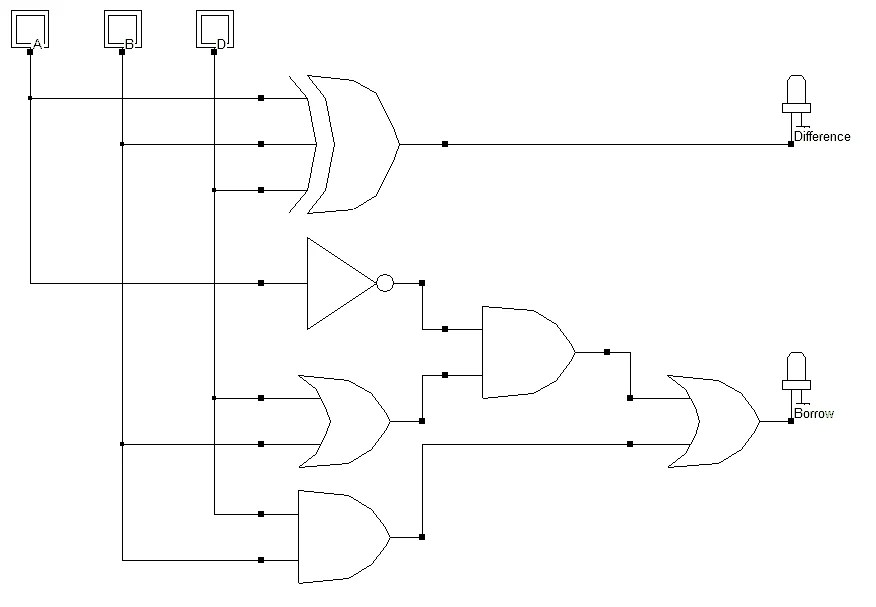View Course Path

# VHDL code for full subtractor using behavioral method – full code & explanation

In this post, we will take a look at implementing the VHDL code for full subtractor using behavioral method. We have seen the design for a full subtractor in our digital electronics course. Here, first, we will explain the logic and then the syntax. For the full code, scroll down.

Contents

## Explanation of the VHDL code for full subtractor using behavioral method. How does the code work?

### Logic circuit of the full subtractor### Truth table of the full subtractor

 A B D DIFFERENCE BORROW 0 0 0 0 0 0 0 1 1 1 0 1 0 1 1 0 1 1 0 1 1 0 0 1 0 1 0 1 0 0 1 1 0 0 0 1 1 1 1 1

In the VHDL code for full adder post, we made the use of if-else commands. Here, we will make use of if-elsif commands.

## VHDL code for full subtractor using behavioral method

```library IEEE;

use IEEE.STD_LOGIC_1164.ALL;

use IEEE.STD_LOGIC_ARITH.ALL;

use IEEE.STD_LOGIC_UNSIGNED.ALL;

entity FULLSUBTRACTOR_BEHAVIORAL_SOURCE is

Port ( A : in  STD_LOGIC_VECTOR (2 downto 0);

Y : out  STD_LOGIC_VECTOR (1 downto 0));

end FULLSUBTRACTOR_BEHAVIORAL_SOURCE;

architecture Behavioral of FULLSUBTRACTOR_BEHAVIORAL_SOURCE is

begin

process (A)

begin

if (A = "001" or A = "010" or A = "111") then

Y <= "11";

elsif (A = "011") then

Y <= "01";

elsif (A = "100") then

Y <= "10";

else

Y <= "00";

end if;

end process;

end Behavioral;```

## Testbench

```library IEEE;
use IEEE.STD_LOGIC_1164.ALL;
use IEEE.STD_LOGIC_ARITH.ALL;
use IEEE.STD_LOGIC_UNSIGNED.ALL;

entity full_sub_tb is
end entity;

architecture tb of full_sub_tb is
component FULLSUBTRACTOR_BEHAVIORAL_SOURCE is
Port ( A : in STD_LOGIC_VECTOR (2 downto 0);
Y : out STD_LOGIC_VECTOR (1 downto 0));
end component;

signal A: STD_LOGIC_VECTOR(2 downto 0);
signal Y: STD_LOGIC_VECTOR(1 downto 0);

begin

uut: FULLSUBTRACTOR_BEHAVIORAL_SOURCE port map(
A => A, Y => Y);

stim:process
begin

A <= "000";
wait for 20 ns;

A <= "001";
wait for 20 ns;

A <= "010";
wait for 20 ns;

A <= "011";
wait for 20 ns;

A <= "100";
wait for 20 ns;

A <= "101";
wait for 20 ns;

A <= "110";
wait for 20 ns;

A <= "111";
wait for 20 ns;
wait;

end process;

end tb;```